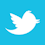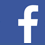A common assumption is differential signals are “equal and opposite.” Is it true?

Periodically, questions about differential trace design rules come up. There is always confusion over whether it is necessary to route differential traces close together, whether a plane needs to be underneath differential traces, or whether to consider differential impedance design rules with differential traces. In one sense, the answers to these questions are difficult, but in another sense they are simple. In fact, if we are not concerned about signal integrity issues, there are no design rules at all. Here is my way of trying to clarify things.

First, what are differential signals, and why are they different? FIGURE 1 illustrates a single (sometimes referred to as a single-ended) trace connecting a driver and a receiver (a) and a differential trace pair (b). Let’s say the signal amplitude (with respect to the reference voltage) in Figure 1a is V = +1. In Figure 1b, there are two signals, V+ = +1 and V- = -1. What the receiver in Figure 2 sees is the difference between these two signals, V+ - V- = +1 – (-1) = +2. The first, and most obvious, difference between the two configurations is that differential signals offer twice the signal level to the receiver. Usually, this translates into twice the signal-to-noise (S/N) level. This is a clear advantage over the single-ended case, and is the primary advantage of differential signals, especially when signal levels are low (as with many sensors).